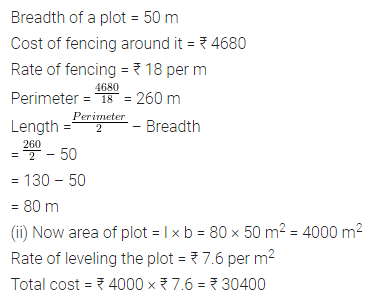ML Aggarwal Class 7 Solutions Chapter 16 Perimeter and Area Ex 16.1 for ICSE Understanding Mathematics acts as the best resource during your learning and helps you score well in your exams.

## ML Aggarwal Class 7 Solutions for ICSE Maths Chapter 16 Perimeter and Area Ex 16.1

Question 1.
ABCD is a square of side 24 cm. EF is parallel to BC and AE = 15 cm. By how much does
(i) the perimeter of AEFD exceed the perimeter of EBCF?
(ii) the area of AEFD exceed the area of EBCF?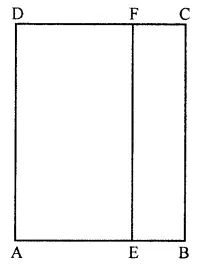Solution:Question 2.
Nagma runs around a rectangular park 180 m long and 120 m wide at the rate of 7.5 km/ hour. In how much time will she complete five rounds?
Solution:Question 3.
The area of a rectangular plot is 540 m2. if its length is 27 m, find its breadth and perimeter.
Solution:Question 4.
The perimeter of a rectangular field is 151 m. If its breadth is 32 m, find its length and area.
Solution: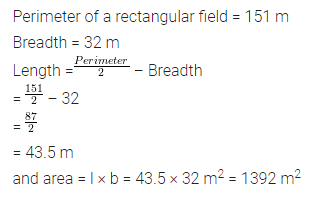Question 5.
The area of a rectangular plot is 340 m2 and its breadth is 17 m. Find the cost of surrounding the plot with a fence at ₹ 5.70 per meter.
Solution: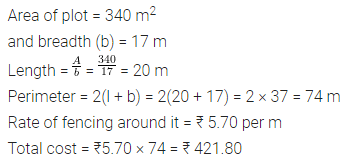Question 6.
The area of a square park is the same as that of a rectangular park. If the side of the square park is 60 m and the length of the rectangular park is 90 m, find the breadth of the rectangular park.
Solution: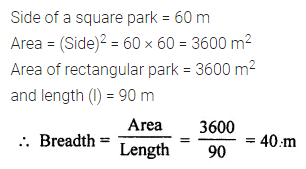Question 7.
A wire is in the shape of a rectangle. Its length is 40 cm and breadth is 22 cm. If the same wire is rebent in the shape of a square, what will be the measure of each side? Also, find which shape encloses more area and by how much?
Solution: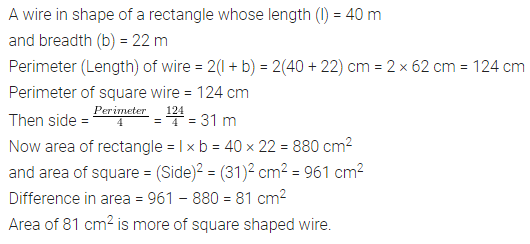Question 8.
A door of breadth 1 m and height 2 m is fitted in a wall. The length of the wall is 4.5 m and the height is 3.6 m. Find the cost of whitewashing the wall, if the rate of whitewashing the wall is ₹ 20 per m2.
Solution:Question 9.
A rectangular park is 45 m long and 30 m wide. A path 2.5 m wide is constructed outside the park. Find the area of the path.
Solution: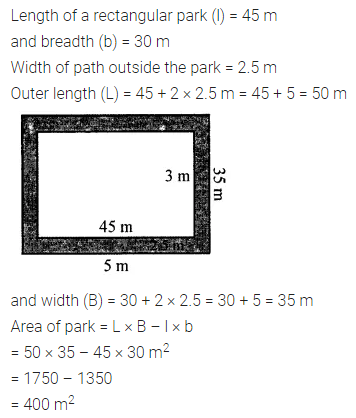Question 10.
A carpet of size 5 m × 2 m has 25 cm wide red border. The inner part of the carpet is blue in colour. Find the area of the blue portion. What is the ratio of the areas of red portion to blue portion?
Solution:Question 11.
A verandah of width 2.25 m is constructed all along outside a room which is 5.5 m long and 4 m wide. Find:
(i) the area of the verandah.
(ii) the cost of cementing the floor of the verandah at the rate of ₹ 200 per m2.
Solution:Question 12.
Two crossroads, each of width 5 m, run at right angles through the centre of a rectangular park of length 70 m and breadth 45 m and parallel to its sides. Find the area of the roads. Also, find the cost of constructing the roads at the rate of ₹ 105 per m2.
Solution: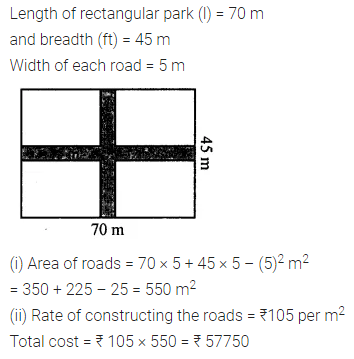Question 13.
A rectangular room is 10 m long and 7.5 m wide. Find the cost of covering the floor with carpet 1.25 m wide at ₹ 250 per metre.
Solution: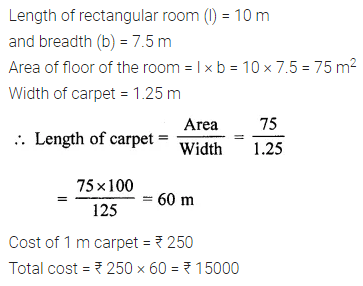Question 14.
Find the cost of flooring a room 6.5 m by 5 m with square tiles of side 25 cm at the rate of ₹ 9.40 per tile.
Solution: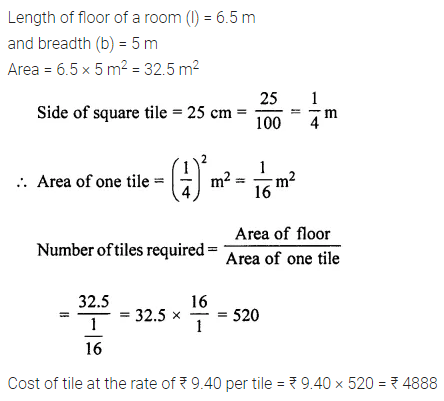Question 15.
The floor of a room is in the shape of a square of side 4.8 m. The floor is to be covered with square tiles of perimeter 1.2 m. Find the cost of covering the floor if each tile costs ₹ 27.
Solution: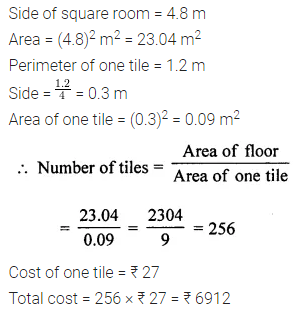Question 16.
A rectangular plot of land is 50 m wide. The cost of fencing the plot at the rate of ₹ 18 per metre is ₹ 4680. Find:
(i) the length of the plot.
(ii) the cost of leveling the plot at the rate of ₹ 7.6 per m2.
Solution: